Courses

# Rotational Motion Practice Level - 1

## 40 Questions MCQ Test | Rotational Motion Practice Level - 1

Description
This mock test of Rotational Motion Practice Level - 1 for Class 11 helps you for every Class 11 entrance exam. This contains 40 Multiple Choice Questions for Class 11 Rotational Motion Practice Level - 1 (mcq) to study with solutions a complete question bank. The solved questions answers in this Rotational Motion Practice Level - 1 quiz give you a good mix of easy questions and tough questions. Class 11 students definitely take this Rotational Motion Practice Level - 1 exercise for a better result in the exam. You can find other Rotational Motion Practice Level - 1 extra questions, long questions & short questions for Class 11 on EduRev as well by searching above.
QUESTION: 1

Solution:
QUESTION: 2

Solution:
QUESTION: 3

### Four identical rods are joined end to end to form asquare. The mass of each rod is M The moment ofinertia of the square about the median line is

Solution:
QUESTION: 4

Two thin discs, each of mass M and radius r m, areattached as shown in fig. to form a rigid body. therotational inertia of this body about an axis perpendicularto the plane of disc B passing through its centre is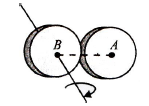Solution:
QUESTION: 5

About which axis moment of inertia in the giventriangular lamina is maximum?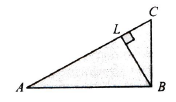Solution:
QUESTION: 6

Figure shows a uniform solid block of mass M and edgelengths a,b and c. Its M.I. about an axis through oneedge and perpendicular (as shown) to the large face ofthe block is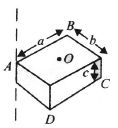Solution:
QUESTION: 7

Figure shows a thin metallic triangular sheet ABC. Themass of the sheet is M. The moment of inertia of thesheet about side AC is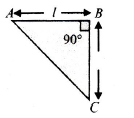Solution:
QUESTION: 8

A square plate of edge a/2 is cut out from a uniform square plate of edge 'a' as shown in fig. The mass of the remaining portion is M. The moment of inertia of the shaded portion about an axis passing through 'O' (centre of the square of side a) and perpendicular to the plane of plate is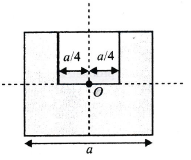Solution:
QUESTION: 9

A uniform ball of radius r rolls without slipping downfrom the top of a sphere of radius R. The angular velocity of the ball when it breaks from the sphere is

Solution:
QUESTION: 10

A solid sphere rests on a horizontal surface. A horizontalimpulse is applied at height h from centre. The spherestarts rolling just after the application of impulse. Theratio h/r will be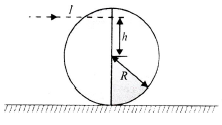Solution:
QUESTION: 11

In Fig. a sphere of radius 2 m rolls on a plank. The acceleration of the sphere and the plank are indicated.The value of α is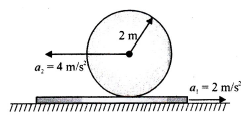Solution:
QUESTION: 12

A ring of radius R is first rotated with an angular velocity ω0  and then carefully placed on a rough horizontal surface. The coefficient of friction betweenthe surface and the ring is μ. Time after which its angular speed is reduced to half is

Solution:
QUESTION: 13

A disc of radius R rolls on a horizontal ground with linear acceleration a and angular acceleration α as shown in Fig. The magnitude of acceleration of point P as shown in the figure at an instant when its linear velocity is v and angular velocity is wω will be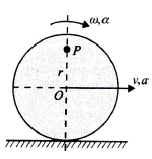Solution:
QUESTION: 14

Two bodies with moments of inertia I1 and I2 (I1 > I2) have equal angular momenta. If their kinetic energies of rotation are E1 and E2 respectively, then

Solution:
QUESTION: 15

Two bodies with moments of inertia I1 and I2 (I1 > I2) have equal angular momenta. If their kinetic energies of rotation are E1 and E2 respectively, then

Solution:
QUESTION: 16

A sphere has to purely roll upwards. At an instant whenthe velocity of sphere is v, frictional force acting on it is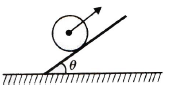Solution:
QUESTION: 17

A uniform disc of mass M and radius R is mounted onan axle supported in frictionless bearings. A light cordis wrapped around the rim of the disc and a steadydownward pull T is exerted on the cord. The angular acceleration of the disc is

Solution:
QUESTION: 18

A uniform rod of mass M and length L is pivoted at oneend such that it can rotate in a vertical plane. There isnegligible friction at the pivot. The free end of the rod isheld vertically above the pivot and then released. The angular acceleration of the rod when it makes an angle θ with the vertical is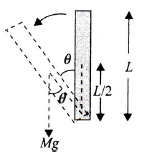Solution:
QUESTION: 19

In Fig. the bar is uniform and weighing 500 N. How large must W be if T1 and T2 are to be equal?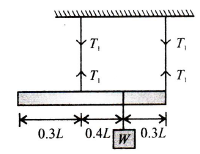Solution:
QUESTION: 20

A smooth uniform rod of length L and mass M has twoidentical bead (1 and 2) of negligible size, each of massm, which can slide freely along the rod. Initially thetwo beads are at the centre of the rod and the systemis rotating with angular velocity ω0 about an axisperpendicular to the rod and is passing through itsmidpoint. There are no external forces when the beads reach the ends of the rod, the angular velocity of thesystem is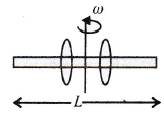Solution:
QUESTION: 21

A uniform thin rod of length I and mass m is hinged ata distance I / 4 from one of the end and released fromhorizontal position as shown in Fig. The angularvelocity of the rod as it passes the vertical position is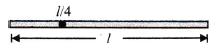Solution:
QUESTION: 22

A disc is rotated about its axis with a certain angularvelocity and lowered gently on a rough inclined planeas shown in Fig. then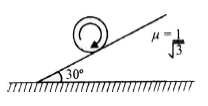Solution:
QUESTION: 23

A child is standing with folded hands at the centre of aplatform rotating about its central axis. The kineticenergy of the system is K. The child now stretches hisarms so that the moment of inertia of the systemdoubles. The kinetic energy of the system now is

Solution:
QUESTION: 24

A ring, cylinder and solid sphere are placed on the topof a rough incline on which the sphere can just rollwithout slipping. When all of them are released at thesame instant from the same position, then

Solution:
QUESTION: 25

If a spherical ball rolls on a table without slipping, the fraction of its total energy associated with rotation is

Solution:
QUESTION: 26

In an experiment with a beam balance, an unknownmass m is Balanced by two known masses of 16 kgand 4 kg as shown in Fig.The value of the unknown mass m is a.

Solution:
QUESTION: 27

A disc of mass m and radius R moves in the x-y plane as shown Fig. The angular momentum of the disc about the origin O at the instant shown is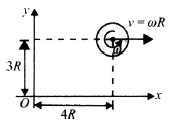Solution:
QUESTION: 28

A spool is pulled horizontally by two equal and oppositeforces as shown in Fig. Which of the followingstatements are correct?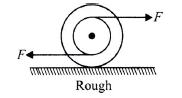Solution:
QUESTION: 29

An impulse J = mv at one end of a stationary uniformfrictionless rod of mass m and length / which is free to rotate in a gravity free space. The impact is elastic. Instantaneous axis of rotation of the rod will pass through

Solution:
QUESTION: 30

A solid sphere of mass m is lying at rest on a rough horizontal surface. The coefficient of friction between the ground and sphere is μ. The maximum value of F,so that the sphere will not slip, is equal to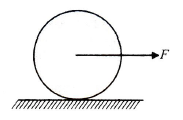Solution:
QUESTION: 31

A sphere is moving towards +ve x-axis with a velocityv and rotates clockwise with angular speed ω as shownin Fig. such that vc >ω R. The instantaneous axis of rotation will be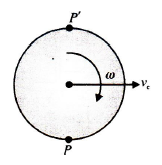Solution:
QUESTION: 32

A cylinder of height H and diameter H/4 is kept on africtional turntable as shown in Fig. The axis of thecylinder is perpendicular to the surface of the tableand the distance of axis of the cylinder is 2H from thecentre of the table. The angular speed of the turntableat which the cylinder will start toppling (assume thatfriction is sufficient to prevent slipping) is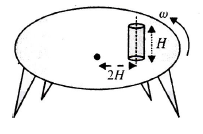Solution:
QUESTION: 33

A uniform rod AB of length I and mass m hangs frompoint A in — a car moving with velocity vo on an inclinedplane as shown in Fig. The rod can rotate in verticalplane about the axis at point A. If the car suddenlystops, the angular speed with which the rod startsrotating is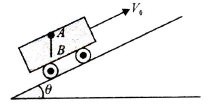Solution:
QUESTION: 34

A uniform rod of mass m, length I rests on a smoothhorizontal surface. Rod is given a sharp horizontalimpulse p perpendicular to the rod at a distance l/4from the centre. The angular velocity of the rod will be

Solution:
QUESTION: 35

A thin rod of length 4/ and mass 4m is bent at thepoints as shown in Fig. What is the moment of inertiaof the rod about the axis passing through point O andperpendicular to the plane of the paper.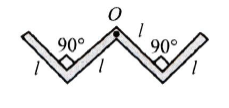Solution:
QUESTION: 36

A uniform circular disc of radius r is placed on a roughhorizontal surface and given a linear velocity v0 andangular velocity ω0  as shown. The disc comes to restafter moving some distance to the right. It follows that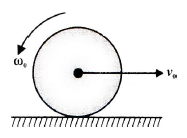Solution:
QUESTION: 37

A smooth inclined plane is fixed in a car acceleratingat a = g tan θ . If the sphere is set pure rolling on theincline, then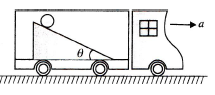Solution:
QUESTION: 38

A cube of side a and mass m is to be tilted at point A by applying a force F as shown in Fig. The minimum force required is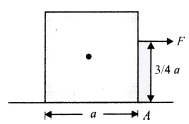Solution:
QUESTION: 39

A ladder of length / and mass m is placed against a smooth vertical wall, but the ground is not smooth.Coefficient of friction between the ground and the ladder is μ. The angle q at which the ladder will stay inequilibrium is

Solution:
QUESTION: 40

A small solid sphere of radius r rolls down an incline without slipping which ends into a vertical loop of radius R. Find the height above the base so that it just loop

Solution: